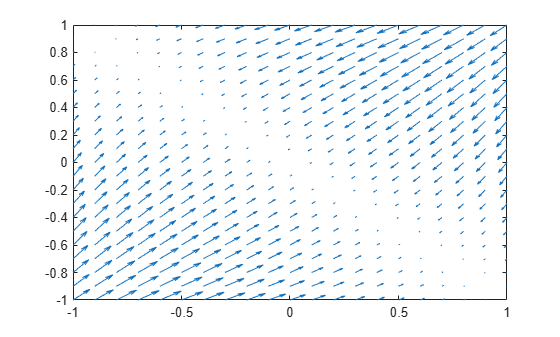## Syntax

``g = gradient(f,v)``
``g = gradient(f)``
``gM = gradient(fM,vM)``

## Description

example

````g = gradient(f,v)` finds the gradient vector of the scalar function `f` with respect to vector `v` in Cartesian coordinates. The input `f` is a function of symbolic scalar variables and the vector `v` specifies the scalar differentiation variables.```
````g = gradient(f)` finds the gradient vector of the scalar function `f` with respect to a vector constructed from all symbolic scalar variables found in `f`. The order of variables in this vector is defined by `symvar`.```
````gM = gradient(fM,vM)` finds the gradient vector of the scalar function `fM` with respect to vector `vM` in Cartesian coordinates. The input function `fM` is a function of symbolic matrix variables and the vector `vM` is a symbolic matrix variable of size `1`-by-`N` or `N`-by-`1`. (since R2021b)```

## Examples

collapse all

The gradient of a scalar function `f` with respect to the vector `v` is the vector of the first partial derivatives of `f` with respect to each element of `v`.

Find the gradient vector of `f(x,y,z)` with respect to vector `[x,y,z]`. The gradient is a vector with these components.

```syms x y z f(x,y,z) = 2*y*z*sin(x) + 3*x*sin(z)*cos(y); gradient(f,[x,y,z])```
```ans(x, y, z) =  $\left(\begin{array}{c}3 \mathrm{cos}\left(y\right) \mathrm{sin}\left(z\right)+2 y z \mathrm{cos}\left(x\right)\\ 2 z \mathrm{sin}\left(x\right)-3 x \mathrm{sin}\left(y\right) \mathrm{sin}\left(z\right)\\ 2 y \mathrm{sin}\left(x\right)+3 x \mathrm{cos}\left(y\right) \mathrm{cos}\left(z\right)\end{array}\right)$```

Find the gradient of a function `f(x,y)`, and plot it as a quiver (velocity) plot.

Find the gradient vector of `f(x,y)` with respect to vector `[x,y]`. The gradient is vector `g` with these components.

```syms x y f = -(sin(x) + sin(y))^2; g = gradient(f,[x,y])```
```g =  $\left(\begin{array}{c}-2 \mathrm{cos}\left(x\right) \left(\mathrm{sin}\left(x\right)+\mathrm{sin}\left(y\right)\right)\\ -2 \mathrm{cos}\left(y\right) \left(\mathrm{sin}\left(x\right)+\mathrm{sin}\left(y\right)\right)\end{array}\right)$```

Now plot the vector field defined by these components. MATLAB® provides the `quiver` plotting function for this task. The function does not accept symbolic arguments. First, replace symbolic variables in expressions for components of `g` with numeric values. Then use `quiver`.

```[X, Y] = meshgrid(-1:.1:1,-1:.1:1); G1 = subs(g(1),[x y],{X,Y}); G2 = subs(g(2),[x y],{X,Y}); quiver(X,Y,G1,G2)```Since R2021b

Use symbolic matrix variables to define a matrix multiplication that returns a scalar.

```syms X Y [3 1] matrix A = Y.'*X```
`A = ${Y}^{\mathrm{T}} X$`

Find the gradient of the matrix multiplication with respect to $X$.

`gX = gradient(A,X)`
`gX = $Y$`

Find the gradient of the matrix multiplication with respect to $Y$.

`gY = gradient(A,Y)`
`gY = $X$`

Since R2021b

Find the gradient of the multivariable function

`$f\left(x\right)={\mathrm{sin}}^{2}\left({x}_{1,1}\right)+{\mathrm{sin}}^{2}\left({x}_{1,2}\right)+{\mathrm{sin}}^{2}\left({x}_{1,3}\right)$`

with respect to the vector $x=\left[{x}_{1,1},{x}_{1,2},{x}_{1,3}\right]$.

Use symbolic matrix variables to express the function $f$ and its gradient in terms of the vector $x$.

```syms x [1 3] matrix f = sin(x)*sin(x).'```
`f = $\mathrm{sin}\left(x\right) {\mathrm{sin}\left(x\right)}^{\mathrm{T}}$`
`g = gradient(f,x)`
`g = $2 \left(\mathrm{cos}\left(x\right)\odot {\mathrm{I}}_{3}\right) {\mathrm{sin}\left(x\right)}^{\mathrm{T}}$`

To show the gradient in terms of the elements of $x$, convert the result to a vector of symbolic scalar variables using `symmatrix2sym.`

`g = symmatrix2sym(g)`
```g =  $\left(\begin{array}{c}2 \mathrm{cos}\left({x}_{1,1}\right) \mathrm{sin}\left({x}_{1,1}\right)\\ 2 \mathrm{cos}\left({x}_{1,2}\right) \mathrm{sin}\left({x}_{1,2}\right)\\ 2 \mathrm{cos}\left({x}_{1,3}\right) \mathrm{sin}\left({x}_{1,3}\right)\end{array}\right)$```

Alternatively, you can convert $f$ and $x$ to symbolic expressions of scalar variables and use them as inputs to the `gradient` function.

`g = gradient(symmatrix2sym(f),symmatrix2sym(x))`
```g =  $\left(\begin{array}{c}2 \mathrm{cos}\left({x}_{1,1}\right) \mathrm{sin}\left({x}_{1,1}\right)\\ 2 \mathrm{cos}\left({x}_{1,2}\right) \mathrm{sin}\left({x}_{1,2}\right)\\ 2 \mathrm{cos}\left({x}_{1,3}\right) \mathrm{sin}\left({x}_{1,3}\right)\end{array}\right)$```

## Input Arguments

collapse all

Scalar function, specified as a symbolic expression or symbolic function that is a function of symbolic scalar variables.

Data Types: `sym` | `symfun`

Vector with respect to which you find gradient vector, specified as a symbolic vector. By default, `v` is a vector constructed from all symbolic scalar variables found in `f`. The order of variables in this vector is defined by `symvar`.

If `v` is a scalar, `gradient(f,v) = diff(f,v)`. If `v` is an empty symbolic object, such as `sym([])`, then `gradient` returns an empty symbolic object.

Data Types: `sym`

Since R2021b

Scalar function, specified as a symbolic expression that is a function of symbolic matrix variables.

Data Types: `symmatrix`

Since R2021b

Vector with respect to which you find gradient vector, specified as a symbolic matrix variable of size `1`-by-`N` or `N`-by-`1`.

Data Types: `symmatrix`

## Output Arguments

collapse all

Gradient vector, returned as a symbolic expression or symbolic function that is a function of symbolic scalar variables.

Data Types: `sym` | `symfun`

Since R2021b

Gradient vector, returned as a symbolic expression that is a function of symbolic matrix variables.

Data Types: `symmatrix`

collapse all

The gradient vector of f(x) with respect to the vector $x=\left({x}_{1},{x}_{2},\dots ,{x}_{n}\right)$ is the vector of the first partial derivatives of f.

`${\nabla }_{x}f\left(x\right)=\left(\frac{\partial f}{\partial {x}_{1}},\frac{\partial f}{\partial {x}_{2}},\dots ,\frac{\partial f}{\partial {x}_{n}}\right)$`